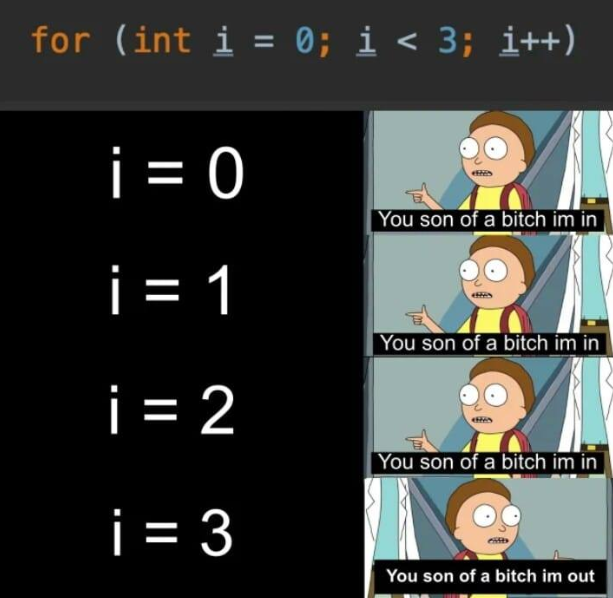# Two Simple Ways to Sum an Array in JavaScriptFor Loop Rick and Morty Meme

## For Loop

`function sumSimpleArray(array) {}`
`function sumSimpleArray(array) {     let sum = 0;}`
`function sumSimpleArray(array) {     let sum = 0;     for(let i = 0; i < array.length; i++) {          sum += array[i];     }     return sum;}`

## Reduce Method

`function sumSimpleArray(array) {     let sum = 0;     const reducer = (accumulator, currentValue) => {          return accumulator + currentValue      }}`
`function sumSimpleArray(array) {     let sum = 0;     const reducer = (accumulator, currentValue) => {          return accumulator + currentValue      }           return array.reduce(reducer)}`
`function sumSimpleArray(array) {     let sum = 0;     return array.reduce((accumulator, currentValue) => {          return accumulator + currentValue      )}}`

Full stack web developer. Frontend and design enthusiast. Avid rock climber and amateur photographer.

## More from Tiffany Kanjanabout

Full stack web developer. Frontend and design enthusiast. Avid rock climber and amateur photographer.